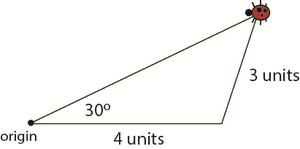Match Fishtank is now Fishtank Learning!

# Geometry

## Objective

Determine if conditions describe a unique triangle, no triangle, or more than one triangle.

## Common Core Standards

### Core Standards

?

• 7.G.A.2 — Draw (freehand, with ruler and protractor, and with technology) geometric shapes with given conditions. Focus on constructing triangles from three measures of angles or sides, noticing when the conditions determine a unique triangle, more than one triangle, or no triangle.

## Criteria for Success

?

1. Given three conditions about a triangle, determine if a unique triangle can be made, and if so, then draw the triangle.
2. Explain why three given conditions about a triangle may result in more than one or no triangles.

## Tips for Teachers

?

• The following materials are needed for this lesson: rulers, protractors, blank paper, and compasses.
• This lesson requires some preparation work for Anchor Problem #1: Using Handout #1, cut out the cards at the bottom of the page. Each pair or group will need a set.
• This article from SERP Poster Problems nicely breaks down the 7.G.2 standard language and is a valuable read prior to the lesson.

#### Remote Learning Guidance

If you need to adapt or shorten this lesson for remote learning, we suggest prioritizing Anchor Problem 2 (benefits from worked example). Find more guidance on adapting our math curriculum for remote learning here.

#### Fishtank Plus

• Problem Set
• Student Handout Editor
• Vocabulary Package

## Anchor Problems

?

### Problem 1

Each group or pair of students gets a few copies of Handout #2 and a set of cards from Handout #1

• Draw three cards and place them in the appropriate places on the Handout #2 form. This represents three conditions about a triangle. Once you’ve placed them on the form, do not change the order.
• Determine if the set of conditions will make one unique triangle, more than one triangle, or no triangle.
• If you can make one unique triangle, draw and label the triangle.
• If more than one or no triangle can be made, be prepared to explain your reasoning.
• Choose another three cards and repeat the activity.

#### References

SERP Poster Problems Triangles to OrderHandout #1 and #2

Triangles to Order from Poster Problems is made available by SERP under the CC BY-NC-SA 4.0 license. Accessed March 11, 2018, 11:57 a.m..

Modified by Fishtank Learning, Inc.

### Problem 2

Two triangles are described below. Determine if each description results in one unique triangle, more than one triangle, or no triangle. Explain your reasoning or draw images to support your conclusion.

• Triangle ${EFG}$  is an isosceles triangle with two angles measuring 35°. Side length ${EG}$ measures 10 cm.
• Triangle ${{PQ}R}$  is an isosceles triangle with ${\angle P}$  and ${\angle Q}$   measuring 22°. Side length ${PQ}$ measures 8 cm.

## Problem Set

?The following resources include problems and activities aligned to the objective of the lesson that can be used to create your own problem set.

?

Starting at the origin, a ladybug walked 4 units east. Then she walked a distance of 3 units in an unknown direction. At that time, she was 30 degrees to the north of her original walking direction.

The diagram shows one possibility for the ladybug’s final location. Find a different final location that is also consistent with the given information, and draw the ladybug there.#### References

Illustrative Mathematics A task related to standard 7.G.A.2

A task related to standard 7.G.A.2, accessed on March 11, 2018, 11:55 a.m., is licensed by Illustrative Mathematics under either the CC BY 4.0 or CC BY-NC-SA 4.0. For further information, contact Illustrative Mathematics.

Modified by Fishtank Learning, Inc.

?